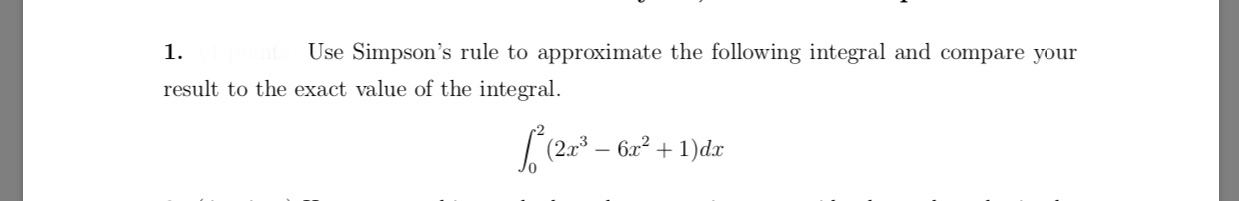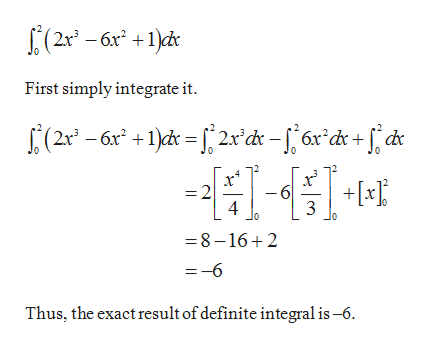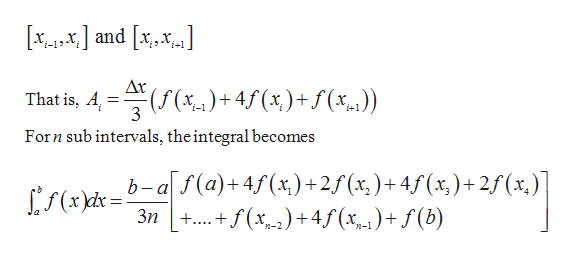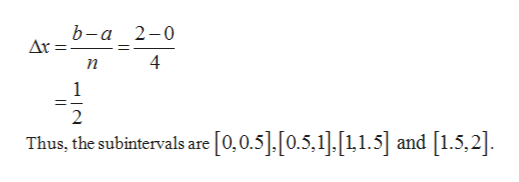Use Simpson's rule to approximate the following integral and compare your1.result to the exact value of the integral.6x21dx

Questionhelp_outlineImage TranscriptioncloseUse Simpson's rule to approximate the following integral and compare your 1. result to the exact value of the integral. 6x21dx fullscreen
Step 1

The integral is given ashelp_outlineImage Transcriptionclose(2x -6x +1) First simply integrate it 2 4 6 + 3 =8-16 2 =-6 Thus, the exact result of definite integral is-6 fullscreen
Step 2

For Simpson’s rule, the area is approximated within two intervalshelp_outlineImage Transcriptioncloseand x,x Ax That is, A 3 )(%.) Forn sub intervals, the integral becomes b-a f(a)+4f(x) + 2f (x, )+4f(x)+ 2f(x.) ...+f(x)4(x)+ f(b) 3n+. fullscreen
Step 3

Obtain the width of eac...help_outlineImage Transcriptioncloseb-a 2-0 n 1 =_ 2 [0,0.5].[0.5.1],11.5] and [1.5,2]. Thus, the subintervals are fullscreen

Want to see the full answer?

See Solution

Want to see this answer and more?

Our solutions are written by experts, many with advanced degrees, and available 24/7

See Solution
Tagged in

Math